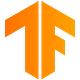# Introducing Neural Structured Learning in TensorFlow

Posted by Da-Cheng Juan (Senior Software Engineer) and Sujith Ravi (Senior Staff Research Scientist)

We are excited to introduce Neural Structured Learning in TensorFlow, an easy-to-use framework that both novice and advanced developers can use for training neural networks with structured signals. Neural Structured Learning (NSL) can be applied to construct accurate and robust models for vision, language understanding, and prediction in general.

Many machine learning tasks benefit from using structured data which contains rich relational information among the samples. For example, modeling citation networks, Knowledge Graph inference and reasoning on linguistic structure of sentences, and learning molecular fingerprints all require a model to learn from structured inputs, as opposed to just individual samples. These structures can be explicitly given (e.g., as a graph), or implicitly inferred (e.g., as an adversarial example). Leveraging structured signals during training allows developers to achieve higher model accuracy, particularly when the amount of labeled data is relatively small. Training with structured signals also leads to more robust models. These techniques have been widely used in Google for improving model performance, such as learning image semantic embedding.

Neural Structured Learning (NSL) is an open source framework for training deep neural networks with structured signals. It implements Neural Graph Learning, which enables developers to train neural networks using graphs. The graphs can come from multiple sources such as Knowledge graphs, medical records, genomic data or multimodal relations (e.g., image-text pairs). NSL also generalizes to Adversarial Learning where the structure between input examples is dynamically constructed using adversarial perturbation.

NSL allows TensorFlow users to easily incorporate various structured signals for training neural networks, and works for different learning scenarios: supervised, semi-supervised and unsupervised (representation) settings.

# How Neural Structured Learning (NSL) Works

In Neural Structured Learning (NSL), the structured signals─whether explicitly defined as a graph or implicitly learned as adversarial examples─are used to regularize the training of a neural network, forcing the model to learn accurate predictions (by minimizing supervised loss), while at the same time maintaining the similarity among inputs from the same structure (by minimizing the neighbor loss, see the figure above). This technique is generic and can be applied on arbitrary neural architectures, such as Feed-forward NNs, Convolutional NNs and Recurrent NNs.

# Create a Model with Neural Structured Learning (NSL)

With NSL, building a model to leverage structured signals becomes easy and straightforward. Given a graph (as explicit structure) and training samples, NSL provides a tool to process and combine these examples into TFRecords for downstream training :

`python pack_nbrs.py --max_nbrs=5 \labeled_data.tfr \unlabeled_data.tfr \graph.tsv \merged_examples.tfr `

Next, NSL provides APIs to “wrap around” the custom model to consume the processed examples and enable graph regularization. Let’s directly take a look at the code example.

`import neural_structured_learning as nsl# Create a custom model — sequential, functional, or subclass. base_model = tf.keras.Sequential(…)# Wrap the custom model with graph regularization. graph_config = nsl.configs.GraphRegConfig( neighbor_config=nsl.configs.GraphNeighborConfig(max_neighbors=1)) graph_model = nsl.keras.GraphRegularization(base_model, graph_config)# Compile, train, and evaluate. graph_model.compile(optimizer=’adam’,  loss=tf.keras.losses.SparseCategoricalCrossentropy(), metrics=[‘accuracy’]) graph_model.fit(train_dataset, epochs=5) graph_model.evaluate(test_dataset)`

With less than 5 additional lines (yes, including the comment!), we obtain a neural model that leverages graph signals during training. Empirically, using a graph structure allows models to be able to train with less labeled data without losing much accuracy (for example, 10% or even 1% of the original supervision).

# What if No Explicit Structure is Given?

What if the explicit structure (such as graphs) is not available or not given as inputs? NSL provides tools for developers to construct graphs from raw data; alternatively, NSL also provides APIs to “induce” adversarial examples as implicit structured signals. Adversarial examples are constructed to intentionally confuse the model一training with such examples usually results in models that are robust against small input perturbations. Let’s take a look at the code example below to see how NSL enables training with adversarial examples.

`import neural_structured_learning as nsl# Create a base model — sequential, functional, or subclass. model = tf.keras.Sequential(…)# Wrap the model with adversarial regularization. adv_config = nsl.configs.make_adv_reg_config(multiplier=0.2, adv_step_size=0.05) adv_model = nsl.keras.AdversarialRegularization(model, adv_config=adv_config)# Compile, train, and evaluate. adv_model.compile(optimizer=’adam’, loss=’sparse_categorical_crossentropy’, metrics=[‘accuracy’]) adv_model.fit({‘feature’: x_train, ‘label’: y_train}, epochs=5) adv_model.evaluate({‘feature’: x_test, ‘label’: y_test})`

With less than 5 additional lines (again, including the comment), we obtain a neural model that trains with adversarial examples providing an implicit structure. Empirically, models trained without adversarial examples suffer from significant accuracy loss (e.g., 30% lower) when malicious yet not human-detectable perturbations are added to inputs.

Please visit https://www.tensorflow.org/neural_structured_learning/, and try out NSL today!

# Acknowledgements

We would like to acknowledge core contributions from Chun-Sung Ferng, Arjun Gopalan, Allan Heydon, Yicheng Fan, Chun-Ta Lu, Philip Pham and Andrew Tomkins. We also want to thank Daniel ‘Wolff’ Dobson and Karmel Allison for their technical suggestions, Mark Daoust, Billy Lamberta and Yash Katariya for their help in creating the tutorials, and Google Expander team for their feedback.

## TensorFlow

TensorFlow is an end-to-end open source platform for…

## TensorFlow

TensorFlow is an end-to-end open source platform for machine learning.

Written by

## TensorFlow

TensorFlow is a fast, flexible, and scalable open-source machine learning library for research and production.## TensorFlow

TensorFlow is an end-to-end open source platform for machine learning.